# Density Matrix

(redirected from Density operator)

## Density matrix

A matrix which is constructed as the most general statistical description of the states of a many-particle quantum-mechanical system. The state of a quantum system is described by a normalized wave function ψ(x, t) [where x stands for all coordinates of the system, and t for the time], which satisfies the Schrödinger equation (1), where H is

(1)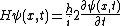the hamiltonian of the system, and &hstrok; is Planck's constant divided by 2π. Furthermore, ψ(x, t) may be expanded in terms of a complete orthonormal set {&phiv;(x)}, as in Eq. (2). Then, the
(2)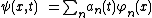(3)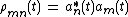density matrix is defined by Eq. (3), and this density matrix describes a pure state. Examples of pure states are a beam of polarized electrons and the photons in a coherent beam emitted from a laser. See Laser, Quantum mechanics

In quantum statistics, one deals with an ensemble of N systems which have the same hamiltonian. If the αth member of the ensemble is in the state ψα in Eq. (4), the density matrix is defined as the ensemble average, Eq. (5).

(4)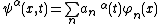(5)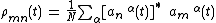In general, this density matrix describes a mixed state, for example, a beam of unpolarized electrons or the photons emitted from an incoherent source such as an incandescent lamp. The pure state is a special case of the mixed state when all members of the ensemble are in the same state. See Statistical mechanics

## Density Matrix

an operator by means of which it is possible to calculate the average value of any physical quantity in quantum statistical mechanics and, in particular, in quantum mechanics. A density matrix describes a system’s state based on an incomplete set (incomplete in terms of quantum mechanics) of data on the system (seeMIXED STATE).

## density matrix

[′den·səd·ē ′mā·triks]
(quantum mechanics)
A matrix ρ mn describing an ensemble of quantum-mechanical systems in a representation based on an orthonormal set of functions φ n; for any operator G with representation Gmn, the ensemble average of the expectation value of G is the trace of ρ G.
References in periodicals archive ?
Nagy, "Isometries and relative entropy preserving maps on density operator," Linear and Multilinear Algebra, vol.
In the mathematical framework of information theory, density operators (quantum states) are positive operators with trace 1 on complex separable Hilbert space H, and the set of all density operators is denoted by S(H) on H.
The density matrix can be constructed through the density operator, [[rho].sup.[conjunction]].
In the statistical point of view, a state of quantum ensemble is described by a density operator (or statistical operator) ([[??].sub.u]; the corresponding matrix is called a density matrix.
By choosing an orthonormal basis (|[u.sub.j]>), one may resolve the density operator into the density matrix, whose elements are
It can be used for a wide range of open quantum systems to solve the master equation by unraveling the density operator evolution into individual stochastic trajectories in Hilbert space .
His topics include the one-particle relativistic distribution function, curved space-time and cosmology, the density operator, applications to nuclear matter, and the relativistic Fermi liquid.
Such density excitations and density quasiparticles appear because of the remaining density operator term that describes atoms above the condensate, a term which was neglected by Bogoliubov .
Using very detailed exercises (with solutions) and samples, and a conversational style, he describes qubits and quantum states, matrices and operations, tensor products, the density operator, quantum measurement theory, entanglement, quantum gates and circuits, quantum algorithms, applications of entanglement including teleportation and super-dense coding, quantum cryptography, quantum noise and error correction, tools of quantum information theory, adiabatic quantum computation and cluster state quantum computing.
Given any density operator [D.sub.S] for S, the probability that any given proposition will be found to be true on measurement is calculated by inserting the corresponding projection operator P into the formula Tr(PDs).
He focuses on methodologies and analysis of key applications that underlie physical, chemical and biological phenomena in complex systems, reviewing the relevant mathematics, then covering quantum dyanics using the time-dependent Schrodinger equation, quantum electrodynamics and matter-radiation field interaction, solids and their interfaces, liquids, and methods including time correlation functions, stochastic processes and equations of motion, quantum relocation processes, the quantum mechanical density operator, linear response theory, and the Spin-Boson model.
The main focus of OMNES will be centered on exploring the following three interconnected pathways: Most importantly, we shall develop a general framework for exact solutions of non-equilibrium integrable quantum many-body models, in particular the steady states and relaxation modes, and develop quantum integrability methods for non-equilibrium many-body density operators. Fundamentally new concepts which are expected to emerge from these studies, relevant beyond the context of boundary-driven/dissipative systems, are novel quasilocal conservation laws of the bulk Hamiltonian dynamics.

Site: Follow: Share:
Open / Close